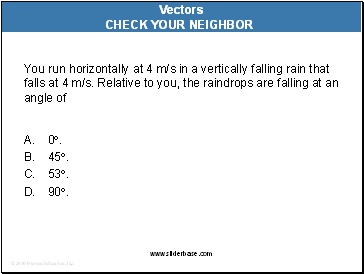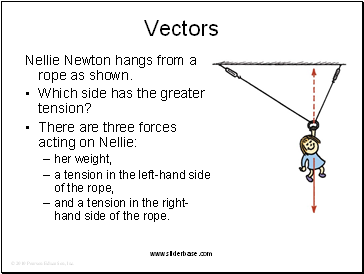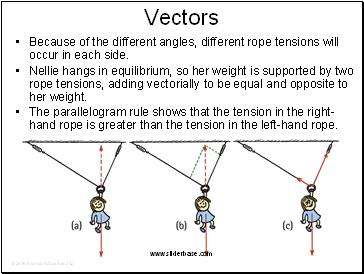# Newton’s third law of motionPage 6

#### WATCH ALL SLIDESYou run horizontally at 4 m/s in a vertically falling rain that falls at 4 m/s. Relative to you, the raindrops are falling at an angle of

A. 0.

45.

53.

90.

Vectors

CHECK YOUR NEIGHBOR

Slide 40You run horizontally at 4 m/s in a vertically falling rain that falls at 4 m/s. Relative to you, the raindrops are falling at an angle of

A. 0.

45.

53.

90.

Explanation: The horizontal 4 m/s and vertical 4 m/s combine by the parallelogram rule to produce a resultant of 5.6 m/s at 45.

Vectors

CHECK YOUR ANSWER

Slide 41Vectors

Nellie Newton hangs from a rope as shown.

Which side has the greater tension?

There are three forces acting on Nellie:

her weight,

a tension in the left-hand side of the rope,

and a tension in the right-hand side of the rope.

Slide 42Vectors

Because of the different angles, different rope tensions will occur in each side.

Nellie hangs in equilibrium, so her weight is supported by two rope tensions, adding vectorially to be equal and opposite to her weight.

The parallelogram rule shows that the tension in the right-hand rope is greater than the tension in the left-hand rope.

Go to page:
1  2  3  4  5  6

## Last added presentations

© 2010-2019 powerpoint presentations